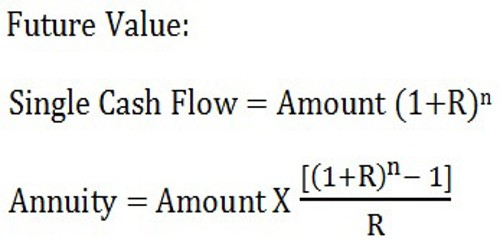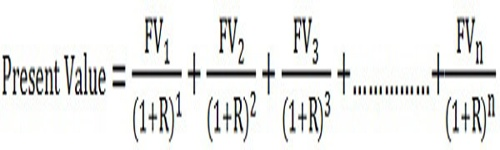Definition of Compounding –

When interest is earned on the principal and the entire amount is again invested to earn more money that is called compounding. Usually, when money is invested with a bank or a credit firm, they compound interest in a fixed time period. This could be weekly, monthly, half-yearly, or annually.

Compounding is the method used in finding out the future value of the present investment. The future value can be computed by applying the compound interest formula which is as under:Where:

n = number of years

R = Rate of return on investment

The best way to understand this process is by example.

In this illustration let us suppose that we have invested a sum of \$1000 with an interest rate of 10% per annum.

So in two years’ time, we would have earned another \$200 bringing us to a total sum of \$1200 without compounding our interests.

However, if we were compounding the interest on the amount annually, we would make more money. In the first year, we would have earned \$100 bringing us to a total amount of \$1100. By compounding interest yearly, the earned \$100 will be added to the principal amount and so in the second year, we will earn 10% interest on \$1100 which is \$110.

Definition of Discounting –

For an investment to be valuable, it must give the investor returns in the future. Calculating the value of those future cash flows in terms of the present by using a discount factor is called discounting. This is an important process because it can be used to compare the possibilities arising from multiple options. It is a vital part of deciding where to invest capital.

Actually, Discounting is the process of converting the future amount into its Present Value. The discounting technique helps to ascertain the present value of future cash flows by applying a discount rate. The following formula is used to know the present value of a future sum:Where:

1,2,3,…..n represents future years

FV = Cash flows generated in different years,

R = Discount Rate

In determining the discounted value of an investment, we must first calculate the returns that it will bring and the time period in which that will occur. Then by applying the formula for discounting the discount factor for the time periods can be calculated. The final step of this process is to multiply the first result by the second and that brings us to the present value of our investment.

The formula for discounting is given below:

Dn = 1/(1+r)n

Here Dn stands for discounting factor. The r is for discounting rate and the n is for the number of time periods being discounted. If the discounting factor is high, then the risk involved with the investment will also be high.

Key Differences Between Compounding and Discounting –

The following are the major differences between compounding and discounting:

• The method uses to know the future value of a present amount is known as Compounding. The process of determining the present value of the amount to be received in the future is known as Discounting.
• Compounding uses compound interest rates while discount rates are used in Discounting.
• Compounding of a present amount means what will we get tomorrow if we invest a certain sum today. Discounting of future sum means, what should we need to invest today to get the specified amount tomorrow.
• The future value factor table is referred to calculate the future value in case of compounding. Conversely, in discounting, the present value can be computed with the help of a Present value factor table.
• In compounding, the present value amount is already specified. On the other hand, the future value is given in the case of discounting.

Compounding and discounting seem like opposing concepts because the former is used to determine the future value of a present investment and the latter is used to determine the present value of future cash flow. However, the common ground they share is that both are extremely useful for those who are looking to invest money.

So, we can say that if we reverse compounding it will become discounting. Compounding Factor table and Discounting Factor table is taken into consideration for the quick calculation of the two. In the table, we will find the factors, concerning different rates and periods. The factor is directly multiplied by the amount to arrive at the present or future value.

Information Sources: